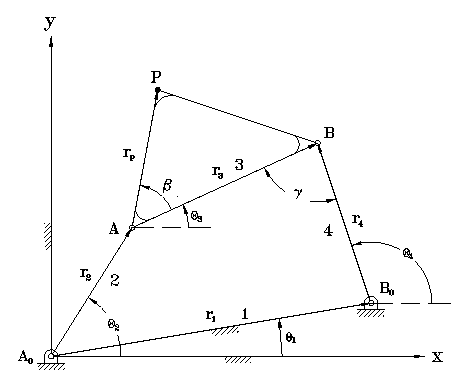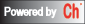# Interactive Four-Bar Linkage Position AnalysisPosition analysis begins with formulating the loop-closure equation for the fourbar mechanism shown below.
```r2+r3=r1+r4	    (1)
```
Incorporating complex numbers,

```r2*exp(i*theta2)+r3*exp(i*theta3)=r1*exp(i*theta1)+r4*exp(i*theta4)	(2)
```

Note link lengths r1,r2,r3, and r4 along with theta1 are constants. Let theta2 be the independent variable, and theta3 and theta4 be the dependent variables. Rearanging the equation, we have

```r3*exp(i*theta3)-r4*exp(i*theta4)=r1*exp(i*theta1)-r2*exp(i*theta2).	(3)
```

Let R1=r3, phi1=theta3, R2=-r4, phi2=theta4, and z=(x3,y3)=r1*exp(i*theta1)-r2*exp(i*theta2). We now have a general complex equation

```R1*exp(i*phi1)+R2*exp(i*phi2)=z.	(4)
```

Angular positions theta3 and theta4 can now be solved for given parameters r1,r2,r3,r4,theta1, and theta2. From equation (4) we obtain

```cos(phi1)=(x3-R2cos(phi2))/R1		(5)
```
```sin(phi1)=(y3-R2sin(phi2))/R1.		(6)
```

Substituting these results into the trig identity sin^2(phi1)+cos^2(phi1)=1 and simplifying we obtain

```y3*sin(phi2)+x3*cos(phi2)=(x3^2+y3^2+R2^2-R1^2)/2*R2. 	(7)
```

From this equation we can obtain formulas for phi1 and phi2

```phi2=atan2(y3,x3)± acos((x3^2+y3^2+R2^2-R1^2)/2*R2*sqrt(x3^2+y3^2))	    (8)
```
```phi1=atan2(sin(phi1),cos(phi1))
=atan2((y3-R2sin(phi2))/R1, (x3-R2cos(phi2))/R1). (9)
```

Similar equations can be derived assuming either theta3 or theta4 is known with the other two angles as parameters.

Please enter link lengths, theta1 and one other known angle to find the other two angles.

Unit Type:
Link lengths (m or ft): r1: r2: r3: r4: rp:
Angles: theta1: beta:

Select and input the known angle (theta2, theta3, or theta4):
value:

Output option:

1. Display angular position
2. Display fourbar position -- Branch Number:
To display the four-bar linkage below on your screen, you need to install Ch Mechanism Toolkit in your local machine.